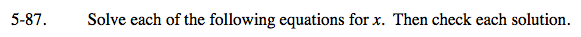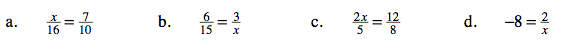### Home > AC > Chapter 5 > Lesson 5.2.3 > Problem5-87

5-87.Set up a proportion to solve for x.

Multiply both sides by 16.

$16\left(\frac{x}{16}\right) = \left(\frac{7}{10}\right)16$

$x = \frac{56}{5}$

x = 11.2

Start by multiplying both sides by x.

Follow the steps in part (a).

Follow the steps in part (b).

x = −0.25